# High School Physics : Introductory Principles

## Example Questions

← Previous 1 3 4 5 6 7 8 9

### Example Question #1 : Understanding Si Units

Which of the following is not an SI unit?

Kilograms

Hertz

Ounces

Meters

Liters

Ounces

Explanation:

The SI unit to measure mass is kilograms. Ounces are also used to measure mass, but are not an SI unit.

Liters are the SI units for volume. Meters are the SI unit for distance. Hertz are the SI unit for frequency.

When in doubt, stick with the metric system. Ounces is an Imperial unit, used almost exclusively in the United States.

### Example Question #2 : Understanding Si Units

Which of the following is an SI unit?

Liters

Miles

Ounces

Pounds

Hours

Liters

Explanation:

Most SI units use the metric system. Liters is the SI unit for volume.

Ounces and pounds are measures of mass, but kilograms are the SI unit for mass. Miles are a measure of distance, but the SI unit for distance is meters. Hours are a measure of time, but the SI unit for time is seconds.

### Example Question #3 : Understanding Si Units

Which of the following is not an SI unit?

Watt

Joule

Calorie

Tesla

Coulomb

Calorie

Explanation:

A calorie is a metric measurement of energy. The standard SI unit for energy is a Joule.

Watts are the SI units for power and teslas are the SI unit for magnetic strength.

### Example Question #4 : Understanding Si Units

Which of the following describes a Newton?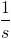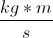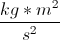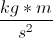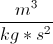Explanation:

A Newton is a unit of force. Remember Newton's second law: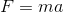.

If you solve this equation purely in terms of units, you can find the equivalent unit for force.Replace force with Newtons, mass with kilograms, and acceleration with meters per square-second.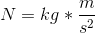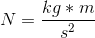Using dimensional analysis, we can find the equivalent units of virtually any measurement.

### Example Question #5 : Understanding Si Units

Which of the following is not an SI unit?

Degrees Celsius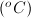Hertz

Mole

Pascal

Ampere

Degrees CelsiusExplanation:

Degrees Celsius is a measurement of temperature, but is not an SI unit. The SI unit for temperature is Kelvin.

Moles are the SI unit for amount of substance in terms of atoms. Ampere is the SI unit for current. Pascal is the SI unit for pressure. Hertz is the SI unit for frequency.

### Example Question #6 : Understanding Si Units

Which of these is not an SI unit?

Amperes

Meters

Seconds

Ohms

Inches

Inches

Explanation:

Inches are a measure of length, but the SI unit for length is meters.

Seconds are the SI units for time, Amperes are the SI units for electrical current, and Ohms are the SI units for resistance.

### Example Question #7 : Understanding Si Units

Which of these is an SI unit?

Acres

Chains

Leagues

Pints

Explanation:

Farads are the SI unit for capacitance.

Leagues and chains are measures of distance, but the SI unit for distance is meters. Acres are a measure of area, the square of a distance. The SI unit for area would be meters-squared. Pints are a unit of volume, but the SI unit for volume is liters.

### Example Question #1 : Understanding Si Units

Which of these is not an SI unit?

Teslas

Sieverts

Newtons

Lumens

Candles

Candles

Explanation:

Candles is a measure of brightness, but the SI units for brightness are lumens.

Teslas are the SI unit for magnetic field strength, Newtons are the SI unit for force, and sieverts are the SI unit for quantifying radiation.

### Example Question #9 : Understanding Si Units

Which of these is an SI unit?

Volt

Hour

Charge

Quart

Knot

Volt

Explanation:

Volts are the SI unit for electromotive force, or electric field strength.

Charge is not a unit, but a property; the SI unit for charge is Amperes. Quarts are a measure of volume, but the SI unit for volume is liters. Hours are a measure of time, but the SI unit for time is seconds. Knots are a measure of velocity, but the SI unit for velocity is meters per second.

### Example Question #10 : Understanding Si Units

Which of these is not an SI unit?

Coulomb

Gallon

Meter

Weber

Watt